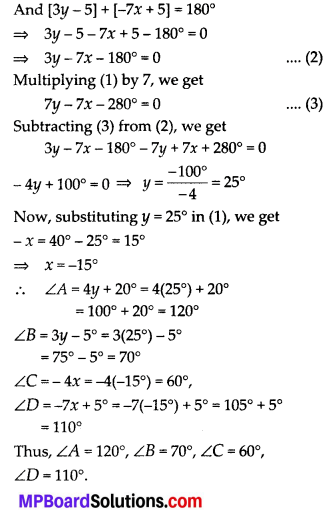# MP Board Class 10th Maths Solutions Chapter 3 Pair of Linear Equations in Two Variables Ex 3.7

In this article, we will share MP Board Class 10th Maths Book Solutions Pair of Linear Equations in Two Variables Ex 3.7 Pdf, These solutions are solved subject experts from the latest edition books.

## MP Board Class 10th Maths Solutions Chapter 3 Pair of Linear Equations in Two Variables Ex 3.7

Question 1.
The ages of two friends Ani and Biju differ by 3 years. Ani’s father Dharam is twice as old as Ani and Biju is twice as old as his sister Cathy. The ages of Cathy and Dharam differ by 30 years. Find the ages of Ani and Biju.
Solution:
Let the age of Ani = x years
and the age of Biju’s = y years
Case I:
y > x
According to 1st condition : y – x = 3 …. (1)
∵ [Age of Ani’s father] = 2[Age of Ani] = 2x yearsSubstituting the value of x in equation (1),
we get y – 21 = 3 ⇒ y = 3 + 21 = 24
∴ Age of Ani = 21 years
Age of Biju = 24 years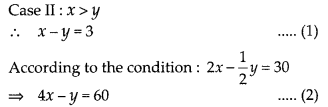Substituting the value of y in equation (1),
we get 19 – y = 3 ⇒ y = 16
∴ Age of Ani = 19 years
Age of Biju = 16 yearsQuestion 2.
One says, “Give me a hundred, friend! I shall then become twice as rich as you”. The other replies, “If you give me ten, I shall be six times as rich as you”. Tell me what is the amount of their (respective) capital? [From the Bijaganita of Bhaskara II]
[Hint: x + 100 = 2(y – 100), y + 10 = 6(x – 10)]
Solution:
Let the capital of 1st friend = ₹ x,
and the capital of 2nd friend = ₹ y
According to the condition,
x + 100 = 2(y -100)
⇒ x + 100 – 2y + 200 = 0
⇒ x – 2y + 300 = 0 … (1)
Also, 6(x – 10) = y + 10 ⇒ 6x – y – 70 = 0 …. (2)
From (1), x = -300 + 2y (3)
Substituting the value of x in equation (2), we get
6[-300 + 2y] – y – 70 = 0
⇒ -1870 + 11y = 0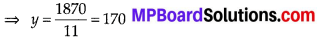Now, Substituting the value of y in equation (3), we get, x = – 300 + 2y
= – 300 + 2(170) = – 300 + 340 = 40
Thus, 1st friend has ₹ 40 and the 2nd friend has ₹ 170.Question 3.
A train covered a certain distance at a uniform speed. If the train would have been 10 km/h faster, it would have taken 2 hours less than the scheduled time. And, if the train were slower by 10 km/h; it would have taken 3 hours more than the scheduled time. Find the distance covered by the train.
Solution:
Let the actual speed of the train = x km/hr
and the actual time taken = y hours
Distance = speed × time
According to 1st condition: (x +10) × (y – 2) = xy
⇒ xy – 2x + 10y – 20 = xy
⇒ 2x – 10y + 20 = 0 … (1)
According to 2nd condition: (x -10) × (y + 3) = xy
⇒ xy + 1ox -10y – 30 = xy
⇒ 3x – 10y – 30 = 0 ….(2)
Using cross multiplication for solving (1) and (2), we get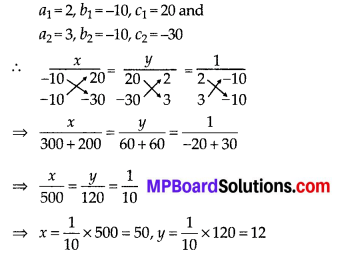Thus, the distance covered by the train = 50 × 12 km = 600 km.Question 4.
The students of a class are made to stand in rows. If 3 students are extra in a row, there would be 1 row less. If 3 students are less in a row, there would be 2 rows more. Find the number of students in the class.
Solution:
Let the number of students = x
and the number of rows = y
∴ Number of students in each row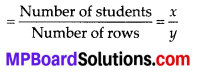Question 5.
In a ∆ABC, ∠C = 3 ∠B = 2(∠A + ∠B). Find the three angles.
Solution:
Sum of angles of a triangle = 180°
∴ ∠A + ∠B + ∠C = 180° … (1)
∵ ∠C = 3∠B = 2(∠A + ∠B) … (2)
From (1) and (2), we have ∠A + ∠B + 2 (∠A + ∠B) = 180°
⇒ ∠A + ∠B + 2∠A + 2∠B = 180°
⇒ ∠A + ∠B = 60° …. (3)
Also, ∠A + ∠B + 3∠B = 180°
⇒ ∠A + 4∠B = 180° ….(4)
Subtracting (3) from (4), we get
∠A + 4∠B – ∠A – ∠B = 180°- 60°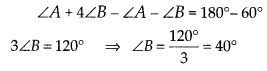Substituting ∠B = 40° in (4) we get,
∠A + 4(40°) = 180°
⇒ ∠A = 180° – 160° = 20°
∴ ∠C = 3∠B = 3 × 40° = 120°
Thus, ∠A = 20°, ∠B = 40° and ∠C = 120°.Question 6.
Draw the graphs of the equations 5x – y = 5 and 3x – y = 3. Determine the co-ordinates of the vertices of the triangle formed by these lines and they-axis.
Solution:
To draw the graph of 5x – y = 5, we get

 x 1 2 0 y 0 5 -5

and for equation 3x – y = 3, we get

 x 2 3 0 y 3 6 -3

Plotting the points (1, 0), (2, 5) and (0, -5), we get a straight line l1. Plotting the points (2, 3), (3, 6) and (0, -3), we get a straight line l2.From the figure, obviously, the vertices of the triangle formed are A(l, 0), B(0, -5) and C(0,-3).

Question 7.
Solve the following pair of linear equations:
(i) px + qy = p – q, qx – py = p + q
(ii) ax + by = c, bx + ay = 1 + c
(iii) $$\frac{x}{a}-\frac{y}{b}$$= 0, ax + by = a2 + b2
(iv) (a – b)x + (a + b)y = a2 – 2ab – b2,
(a + b)(x + y) = a2 + b2
(v) 152x – 378y = -74, – 378x + 152y = -604
Solution: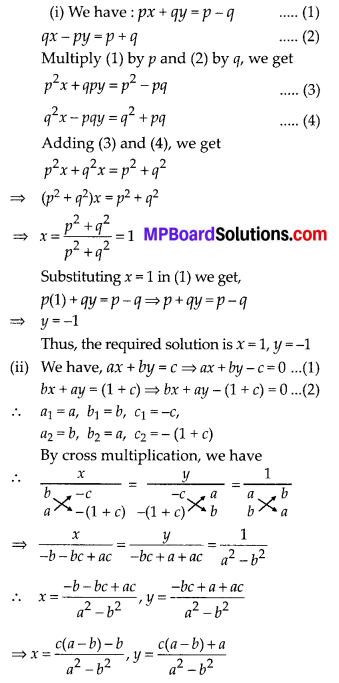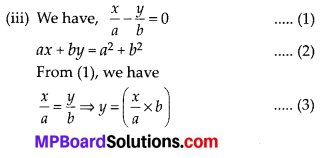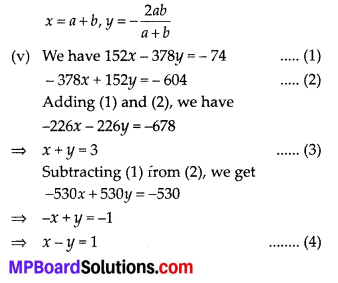Question 8.
ABCD is a cyclic quadrilateral (see figure). Find the angles of the cyclic quadrilateral.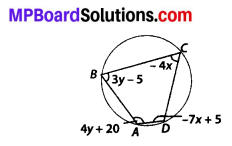Solution: